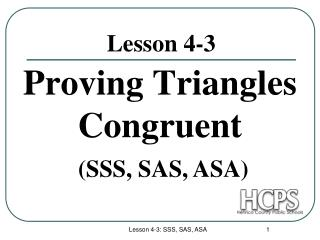DownloadDownload PresentationLesson 4-3

# Lesson 4-3

Download Presentation## Lesson 4-3

- - - - - - - - - - - - - - - - - - - - - - - - - - - E N D - - - - - - - - - - - - - - - - - - - - - - - - - - -
##### Presentation Transcript

1. Proving Triangles Congruent Lesson 4-3 (SSS, SAS, ASA) Lesson 4-3: SSS, SAS, ASA

2. A D F B C E Postulates If the sides of one triangle are congruent to the sides of a second triangle, then the triangles are congruent. SSS Included Angle: In a triangle, the angle formed by two sides is the included angle for the two sides. Included Side: The side of a triangle that forms a side of two given angles. Lesson 4-3: SSS, SAS, ASA

3. Included Angles & Sides Included Angle: * * * Included Side: Lesson 4-3: SSS, SAS, ASA

4. A A D D B C B C F F E E Postulates If two angles and the included side of one triangle are congruent to the two angles and the included side of another triangle, then the triangles are congruent. ASA If two sides and the included angle of one triangle are congruent to the two sides and the included angle of another triangle, then the triangles are congruent. SAS Lesson 4-3: SSS, SAS, ASA

5. Steps for Proving Triangles Congruent • Mark the Given. • Mark … Reflexive Sides/Vertical Angles • Choose a Method. (SSS , SAS, ASA) • List the Parts … in the order of the method. • Fill in the Reasons … why you marked the parts. • Is there more? Lesson 4-3: SSS, SAS, ASA

6. A B @ AB CD 1. @ BC DA 2. @ AC CA 3. C D Problem 1  Step 1: Mark the Given Step 2: Mark reflexive sides SSS Step 3: Choose a Method (SSS /SAS/ASA ) Step 4: List the Parts in the order of the method Step 5: Fill in the reasons Step 6: Is there more? Given Given Reflexive Property SSS Postulate Lesson 4-3: SSS, SAS, ASA

7. Problem 2  Step 1: Mark the Given Step 2: Mark vertical angles SAS Step 3: Choose a Method (SSS /SAS/ASA) Step 4: List the Parts in the order of the method Step 5: Fill in the reasons Step 6: Is there more? Given Vertical Angles. Given SAS Postulate Lesson 4-3: SSS, SAS, ASA

8. X W Y Z Problem 3 Step 1: Mark the Given Step 2: Mark reflexive sides ASA Step 3: Choose a Method (SSS /SAS/ASA) Step 4: List the Parts in the order of the method Step 5: Fill in the reasons Step 6: Is there more? Given Reflexive Postulate Given ASA Postulate Lesson 4-3: SSS, SAS, ASA